## Example Questions

← Previous 1 3

### Example Question #1 : How To Add Exponents

If a2 = 35 and b2 = 52 then a4 + b6 = ?

150,000

140,608

3929

141,833

522

141,833

Explanation:

a4 = a2 * a2  and  b6= b2 * b* b2

Therefore a4 + b6 = 35 * 35 + 52 * 52 * 52 = 1,225 + 140,608 = 141,833

Solve for x.

2+ 2x+1 = 72

3

5

7

4

6

5

Explanation:

8 + 2x+1 = 72

2x+1 = 64

2x+1 = 26

x + 1 = 6

x = 5

### Example Question #3 : How To Add Exponents

Which of the following is eqivalent to 5b – 5(b–1) – 5(b–1) – 5(b–1) – 5(b–1) – 5(b–1) , where b is a constant?

1/5

0

5b–1

5

1

0

Explanation:

We want to simplify 5b – 5(b–1) – 5(b–1) – 5(b–1) – 5(b–1) – 5(b–1) .

Notice that we can collect the –5(b–1) terms, because they are like terms. There are 5 of them, so that means we can write –5(b–1) – 5(b–1) – 5(b–1) – 5(b–1) – 5(b–1) as (–5(b–1))5.

To summarize thus far:

5b – 5(b–1) – 5(b–1) – 5(b–1) – 5(b–1) – 5(b–1) = 5b +(–5(b–1))5

It's important to interpret –5(b–1) as (–1)5(b–1) because the –1 is not raised to the (b – 1) power along with the five. This means we can rewrite the expression as follows:

5b +(–5(b–1))5 = 5b + (–1)(5(b–1))(5) = 5b – (5(b–1))(5)

Notice that 5(b–1) and 5 both have a base of 5. This means we can apply the property of exponents which states that, in general, abac = ab+c. We can rewrite 5 as 51 and then apply this rule.

5b – (5(b–1))(5) = 5b – (5(b–1))(51) = 5b – 5(b–1+1)

Now, we will simplify the exponent b – 1 + 1 and write it as simply b.

5b – 5(b–1+1) = 5b – 5b = 0

### Example Question #4 : How To Add Exponents

If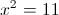, then what does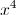equal?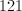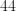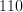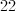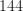Explanation: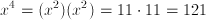### Example Question #1 : Exponents

Simplify.  All exponents must be positive.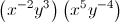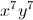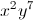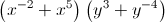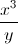Explanation:

Step 1: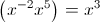Step 2: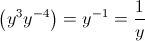Step 3: (Correct Answer):### Example Question #1 : Exponents

Simplify.  All exponents must be positive.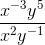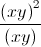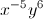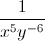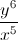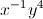Explanation:

Step 1: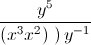Step 2: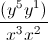Step 3:### Example Question #7 : How To Add Exponents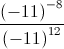Answer must be with positive exponents only.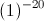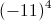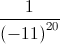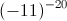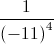Explanation:

Step 1: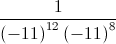Step 2: The above is equal to### Example Question #8 : How To Add Exponents

Evaluate: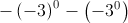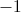Explanation: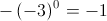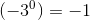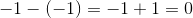### Example Question #9 : How To Add Exponents

Simplify: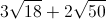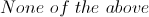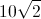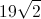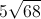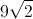Explanation: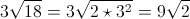Similarly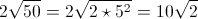So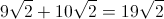### Example Question #71 : Exponents

If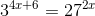, what is the value of?Explanation:

Using exponents, 27 is equal to 33. So, the equation can be rewritten:

34+ 6 = (33)2x

34+ 6 = 36x

When both side of an equation have the same base, the exponents must be equal. Thus:

4x + 6 = 6x

6 = 2x

x = 3

← Previous 1 3